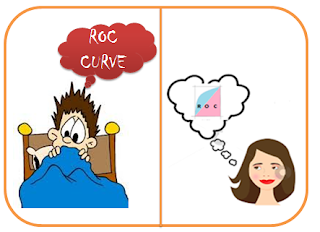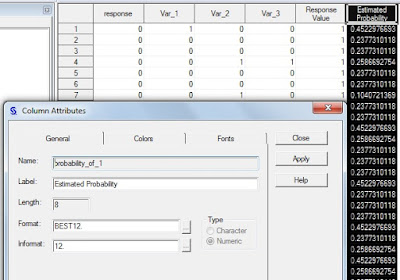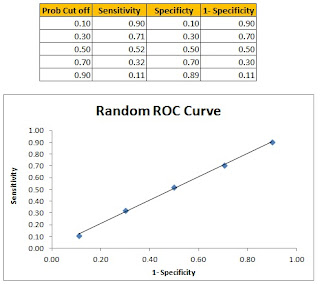## Pages

### An Explicit coverage on ROC curve

One of the most trending interview questions from Logistic Regression is "What do you understand by ROC Curve?". Initially I was scared of any question related to ROC curve in my interviews because the concept was always quite confusing for me. So, I finally decided to battle it out by understanding it to the depth.
Now after understanding it thoroughly, I think it is one of the most interesting topics when it comes to logistic Regression. I am sharing all my understanding on ROC curve through this blog. I hope it would make your learning much more rich.
Before going ahead with ROC curve, I would like you to revise the concept of Sensitivity and Specificity from one of our previous blogs on Logistic Regression at Ask Analytics.

In order to understand the terms Sensitivity and Specificity, please follow the link : Logistic Regression - Part 3 - Result Interpretation, and then come back to this article.

Also there are articles which cover how to build a Logistic Regression :This is I, before and after writing this blog :p

To start with , we have taken a random case study where our Y variable is "response" with value of 1 and 0 where 1 tells that a person defaults and 0 a person doesn't.

I am not going in the details of how I ran the logistic model on my data since you can get all the details on how to build a logistic model on the above listed links. Let us straight away get on to the crux !

After dividing the data into Training and validation sets and running Proc Logistic on the training dataset the model gives  "Result" dataset with a variable 'probability_of_1', which is simply the probability of a customer's defaulting, predicted by our model.

Use the following file to build the model (You can use any other too) :

proc import datafile = "add location\Master_Data.xlsx"
out =  Model_master
dbms = 'xlsx' replace;
sheet = "Data";
run;

Data Training  validation;
set   Model_master;
if ranuni(2) < 0.7 then output Training;
else output  validation;
Run;

Proc Logistic data = Training descending;
Model response = Var_1 Var_2 Var_3
lackfit  ctable  pprob= ( 0 to 1 by 0.1)
outroc = roc_data_training;
output out = result p = probability_of_1;
score data = validation out = validation
outroc = roc_data_validation ;
Run;
Quit;Click to enlarge

Take the values of the response variable (actual Y) and the probability_of_1 (prediction from the model) in an Excel file.I have created a Random Probability column which is nothing but random numbers between 0 and 1. Now, the Random Classification columns at various probability cut-offs that you can see in column D to H (if  random probability, that we just created, is greater than the probability cut-off  then we classify it as 1 else classify it as 0. "1" in the classification means that the person will default whereas "0" in the classification means the person will not default.

Based on these classifications at various probability cut-offs, we had calculated Sensitivity and Specificity ( & 1-  Specificity a well). While we plot the sensitivity and 1-specificity profile for a random model follows a straight 45 degree line, (in case of a large sample). You may see some variance here in the plot since the sample is small, but the ROC curve will be a straight line in case of a random model.

------------------------------------------------*******--------------------------------------------------

This was all about the random model, but what about the probability_of_1 which is actually the probability given by our model. We need not create the sensitivity for this manually ( though we can do so), as in one of the output datasets namely : Roc_data_training and Roc_data_validation.

In the above table we can clearly see that , now the 1- Specificity and Sensitivity remain close to each other. SAS automatically plots the plot of 1- Specificity ( on X axis) and Sensitivity (on Y Axis) at various probability cut-offs that  looks like following chart :

** Often people commit a mistake in reading  the ROC curve. Guys, the cut-off probability increases from the top right to bottom left.

### What are various benefits/usages of ROC Curve ?

Point 1 : Gives goodness of Fit of ModelSince the ROC curve is plotted on the unit scale of Sensitivity and 1-Specifiticity, the area of the   square is 1 and that of the triangle (pink) is 0.5.

This pink triangle is nothing but the area under random probability model. The area under the Model's ROC curve is Blue + Pink shaded area and hence it is generally more than 0.5.

For a model to be good, both Sensitivity and Specificity should be on higher side; so the 1-Specificity should be on lower side. Hence, the model's ROC curve should be closer to Y axis and the blue area, which is the gain that we have received by building the logistic model, should be higher.The area under the ROC curve is same as c-stat, that we get in table of Concordance statistics.  The Larger the c-stats, the better the model and is, thus, a measure of goodness of fit in Logistic regression.

Typically under these area under the curve or c-stats is interpreted as follows:

0.5                 :  Useless model, as good as a random Model
0.6-0.7          :   Below Average Model
0.7-0.75        :   Average to good Model
0.75-0.8        :   Very Good Model

Point 2 : Helps determining the cut-off probability for classification (1/0)

Method 1 is Youden-Index: Consider the probability cut-off  for which (sensitivity+specificity) is maximal.This is more widely used method.Method 2 is Distance Based: The point on ROC curve which is nearest to top left corner of the curve's box should be considered as the optimal probability cut-off point. Mathematically, it is :

Point 3 : One need to keep in mind that the ROC curve should be smooth for it to be a good model.

Point 4: For Validation of the model

The last point that one should keep in mid that the ROC curves of Training and Validation should be close (super imposing) to each other. It these are not close, it means that the model is not holding true on validation dataset.

Enjoy reading our other articles and stay tuned with us.

Kindly do provide your feedback in the 'Comments' Section and share as much as possible.